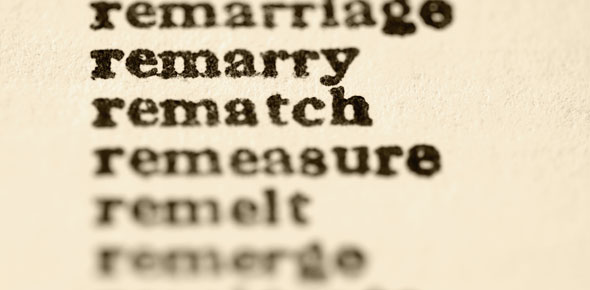# Science

6 QuestionsSettingsThe quiz is my vocabulary that i have to know. It is 6 words so far

Related Topics
• 1.
• A.

A measure of how much matter an object is made of.

• B.

The force of gravity on an object

• C.

Is anything that has mass and volume. Matter exists ordinarily as a solid, a liquid, or a gas.

• 2.
• A.

An amount of three-dismensional space, often used to describe the space that an object takes up.

• B.

A measure of how much matter an object is made of.

• C.

A group of atoms that are held together by covalent bonds so that they move as a single unit.

• 3.
• A.

The force of gravity on an object

• B.

The smallest particle of an element that has the chemical properties of an element.

• C.

Is anything that has mass and volume. Matter exists ordinarily as a solid, a liquid, or a gas.

• 4.
• A.

How loud things are

• B.

The force of gravity on an object

• C.

An amount of three-dismensional space, often used to describe the space that an object takes up.

• 5.
• A.

An amount of three-dismensional space, often used to describe the space that an object takes up.

• B.

The smallest particle of an element that has the chemical properties of an element.

• C.

A T.V. show

• 6.
• A.

A group of atoms that are held together by covalent bonds so that they move as a single unit.

• B.

A measure of how much matter an object is made of.

• C.

A mol that is E que